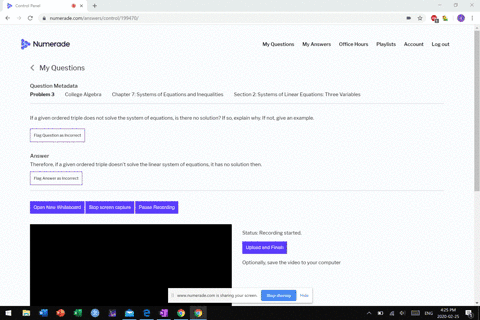Sign up for our free STEM online summer camps starting June 1st!View Summer Courses### If a given ordered triple does not solve the syst…

00:34
MB

Need more help? Fill out this quick form to get professional live tutoring.

Get live tutoring
Problem 2

If a given ordered triple solves the system of equations, is that solution unique? If so, explain why. If not, give an example where it is not unique.

Therefore, if a given ordered triple solves the linear system of equations of three variables the solution is unique.

## Discussion

You must be signed in to discuss.

## Video Transcript

So the question and number two is that order Triple Saul's a system of equations. Um, does that mean that the solution is unique? Okay, so a system of equations in in three variables is the intersection of planes of playing is an infinite flat object. If this solution is unique, which happens often, what that means is that all three planes intersect in a unique point. Life in this one over here. So all of the angles of these different planes have to be a different angles to one another. It is possible, though, for a salute, a solution Thio system of three equations to not be unique. So, for instance, if the planes that are oriented like this any point, that's arm this line right here would represent a solution. So even though you have find one solution, it may not be unique and may not be the only solution there is. So come